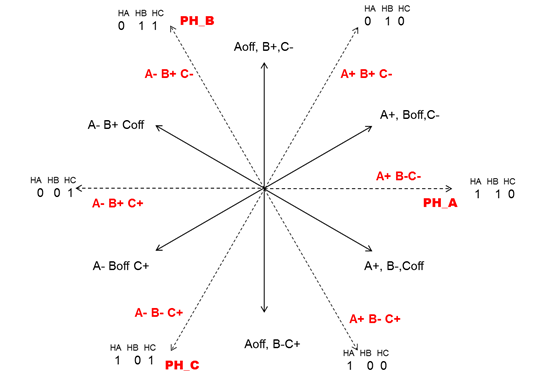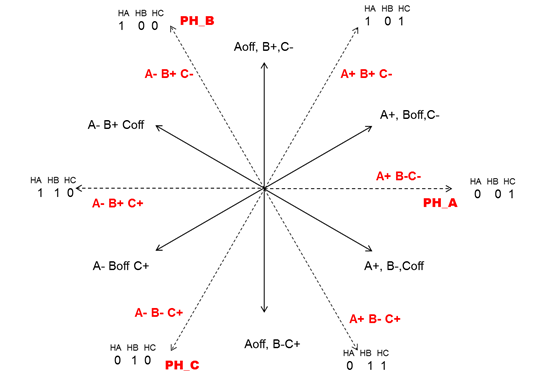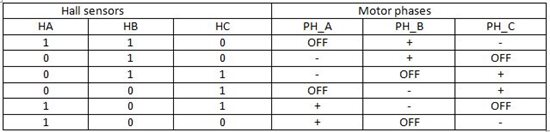# Generate your own commutation table – part 2

In the previous blog, we discussed that deriving the right commutation table plays an important role in controlling 3-phase BLDC motors using hall sensors. As a refresher, here are some surprising facts about the drive system:

• Out of six possible connections between three-phase motor terminals and 3-phase H-bridge outputs, there is only one right connection for both direction of rotation and the remaining 5 are wrong.

• Some think that by swapping two motor terminals direction can be reversed. But, that’s not quite right. Many times motors are able to rotate even using one or two wrong sequences so determining the right commutation sequence is very important to get efficient control.

We also previously covered a method to generate the commutation table based on three-phase motor back EMF waveforms and their relation with respect to hall sensor output. This is based on the assumption that you are able to get the required information from motor manufactures. The reality is that in almost more than 60-70% of cases, the information is not easily available.

If you’re puzzled on how to derive the right commutation sequence and how to get your motor rotating in either direction, please read-on where we’ll cover the steps to generate a commutation table for a given BLDC motor using a DC lab power supply on the actual motor sample without depending upon manufacturer’s data.

The good thing about this approach is that once you follow and understand it, you are able to visualize how a motor actually rotates and subsequently, how to reverse the rotation. The below method assumes that hall sensors are displaced at 120⁰electrically apart from each other.

• Mark three-motor phases as PH_A, PH_B and PH_C as you desire. Do not mark the hall sensors yet.

• Set the current limit of DC power supply to 10-20% of motor rated current.

• Connect PH_A to Positive terminal of DC supply. Connect PH_B and PH_C to negative terminal of power supply. The stator current vector generated inside the motor with this sequence is in phase with magnetic axis of PH_A as shown in figure 3 and 4 by dotted line. The rotor will move to get aligned with PH_A axis.

• Once the rotor becomes stationary, measure the three-hall sensor signals. There are two possibilities of binary code, either with two high level logic (i.e. 1 1 0), or with two low level logic (i.e. 0 0 1), depending upon the motor and hall sensor configuration.  If binary pattern is 1 1 0 (configuration-A), define hall sensor with low logic as HC. If the binary pattern is 0 0 1 (configuration-B), define high logic as HC.

• Apply next excitation with PH_A and PH_B connected to positive terminal and PH_C connected to negative, as shown in figure 3 and 4. This will apply stator current vector which is 60⁰ CCW with respect to PH_A axis. Measure the three hall sensor signals after the rotor comes to a standstill. If the previous pattern resembles configuration-A, define HA as a hall signal which changed from 1 to 0. Similar, if the previous pattern resembles configuration-B, define HA as a hall signal which changed from 0 to 1.  With HA and HC clearly marked, mark the third hall signal as HB.

• Follow figure 3 excitation sequence in CCW direction to complete the vector diagram if your motor and hall configuration resembles to Configuration-A, other-wise follow figure-4.

• It is important to observe that the motor should rotate consecutively in same direction (either CW or CCW) as excitation sequences moves in CCW direction as per figure 3 and 4.

• For simplicity let’s assume that the motor sample resembles configuration-A and with applied CCW sequence of excitation, the motor also rotates in CCW direction as seen by the facing motor shaft. Referring to figure 3, commutation table to rotate the motor in CCW direction is derived from the angle between the stator magnetic field and the rotor flux, which should be 90°. This means that for hall code 1 1 0, commutation sequence Aoff, B+ and C- should be applied. When the motor moves to the next sector and the hall pattern changes to 0 1 0, commutation sequence A-, B+ and Coff should be applied. Following above logic, we get the commutation table 1.Figure 3: Commutation sequence as per configuration-AFigure 4: Commutation sequence as per configuration-BTable 1: Six state commutation table

Using the above method, you can derive the commutation table for reverse direction of rotation. By the way, table 1 is also valid for configuration-B types of motors or hall combinations in reverse (i.e.: clockwise) direction.

Next Step:

TI supplies various motor control kits which support hall sensor interface including the BOOSTXL-DRV8323RS and DRV8353RS-EVM. Grab one and get your motor spinning!